# Precalculus : Application Problems

## Example Questions

2 Next →

### Example Question #11 : Application Problems

The exponential decay of an element is given by the function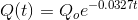where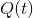is the amount of the element afterdays, and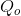is the initial amount of the element. If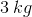of the element are left after four days, how much of the element was there initially, to the nearest tenth of a kilogram?

Possible Answers: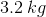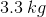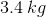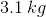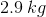Correct answer:Explanation:

To solve for the initial amount, we must use rearrange the equation: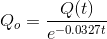We now substitute the values given from the problem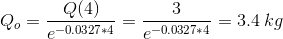### Example Question #158 : Exponential And Logarithmic Functions

There were 240mg of caffeine in the discontinued energy drink. The decay rate for caffeine in the human bloodstream is around 0.14. If Jackie drinks this energy drink around 8PM, how much caffeine will still be in her system at midnight?

Possible Answers: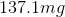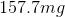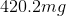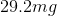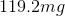Correct answer:Explanation:

Because this is a process taking place in the human body, we should use the exponential decay formula involving e: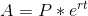where A is the current amount, P is the initial amount, r is the rate of growth/decay, and t is time.

In this case, since the amount of caffeine is decreasing rather than increasing, use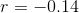. Between 8PM and midnight, 4 hours pass, so use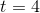. The initial amount of caffeine is given as 240 mg, so use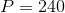.

Now evaluate: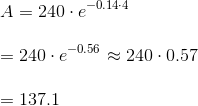### Example Question #151 : Exponential And Logarithmic Functions

Stuff animals were a strange craze of the 90's. A Cat stuff animal with white paws sold for $6 in 1997. In 2015, the Cat will sell for$1015. What has been the approximate rate of growth for these stuff animal felines?

Possible Answers: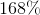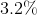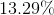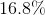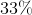Correct answer:Explanation:

Use the formula for exponential growth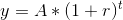where y is the current value, A is the initial value, r is the rate of growth, and t is time. Between 1997 and 2015, 18 years passed, so use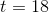. The stuffed animal was originally worth $6, so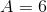. It is now worth$1,015, so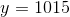.

Our equation is now: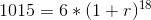divide by 6: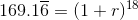take both sides to the power of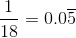: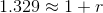subtract 1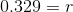As a percent, r is about 33%.

2 Next →

### All Precalculus Resources